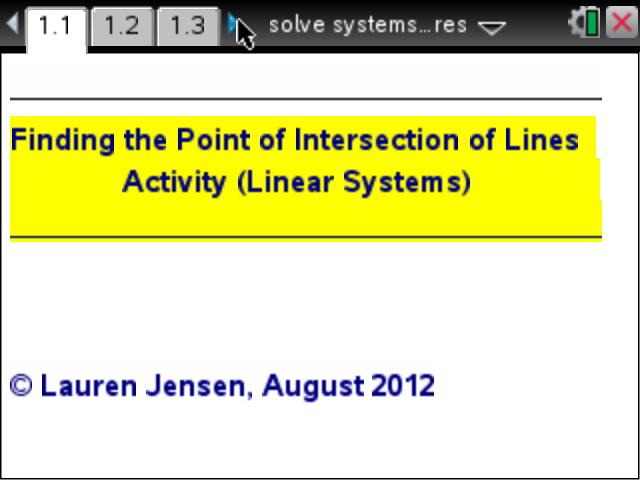# Activities

•• ##### Subject Area

• Math: Algebra I: Systems of Linear Equations
• Math: Precalculus: Systems of Equations
• Math: Algebra II: Systems of Linear Equations and Inequalities

• ##### Author6-8

45 Minutes

• ##### Device
•TI-Nspire™ CX/CX II
• TI-Nspire™ Navigator™
• TI-Nspire™

3.2

## Solving Systems Using Pictures!#### Activity Overview

This activity consists of three separate problems where students have to use systems of linear equations to find the points of intersection of various objects.

Students have to find the linear equations for the sides of a pyramid, set them equal to each other, and find the point that is missing at the top of the pyramid. Students are to follow the same steps for the next two 'problems' which are the tip of a kite and the peaks of three pyramids.